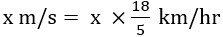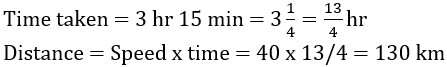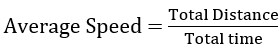# Speed, Time & Distance – Concepts and Formula

## Speed, Time & Distance – Concepts and Formula

One of the most prevalent quantitative aptitude topics asked in government tests is speed, time, and distance. This is one of those topics that students are already aware of before they begin studying for competitive exams.

Although the concepts of speed, time, and distance remain the same, the kind of questions presented in exams may vary.

2-3 word problems related to Speed, Time, and Distance are asked in exams, but candidates may also expect questions about data sufficiency and data interpretation related to Time, Distance, and Speed topic.

So, we are providing you with concepts and formulas of the Speed, Distance, and Time topic to help you prepare properly for the upcoming exams.

When we travel from one point to another, there will be three Quantity that defines this journey – Speed, Distance and Time.

#### Relation between Speed, Distance and Time.Distance = Speed X Time#### Unit of Speed, distance and time

Speed – km/hr , m/s etc
Time – Seconds(s), Hour (h) etc
Distance – meter(m), kilometre(km) etc

Note : If the distance is in Km then time should be in hr and if the distance is in m(meter) then time should be in s ( second)

#### How to convert km/hr into m/s

Let’s suppose we want to convert 9 km/hr into m/s

We know that 1km = 1000 m and 1 h = 3600 s#### How to convert m/s into km/hr

Let’s suppose we want to convert 10 m/s in km/hr
We know that 1km = 1000 m and 1 h = 3600 sQuestion. Convert 18 km/hr into m/s ?
Answer. 18 km/hr = 18 x 5/18 = 5 m/s

Question. Convert 20 m/s into km/hr ?
Answer. 20 m/s = 20 x 18/5 = 72 km/hr

If the ratio of the speeds of A and B is a : b, then the ratio of the times taken by them to cover the same distance isQuestion. Amit takes 21 min to reach his school from his home at the speed of 10 m/s. Next day Amit increases it speed to 15 m/s then how much time he takes to reach the school.
As distance is same in both the cases , Ratio of speed = 10 : 15 = 2:3
So, the ration of time will be = 3 : 2  ,
so, time take in scond case = 21/3 x 2 = 14 min

Alternate method
Distance between school and home = 21 x 60 x 10 = 12600 m
Time taken in scond case = distance / speed = 12600 / 15 = 840 sec
840 sec = 840/60 = 14 min

Alternate method
When distance is equal, we have T1S1 =  T2S2
21 x 10 = T x 15
T = 14 min

Question A person crosses a 600 m long street in 5 minutes. What is his speed in km per hour?
Answer. Speed in m/s = 600/(5 x 60)= 2m/s
Speed in km/hr = 2 x 18/5 = 7.2 km/hr

Question A man covered a distance of 200 km in 4 hours on a bike. How much distance will be covered on a bicycle in 8 hours if he rides the bicycle at one-fifth the speed of the bike?
Answer. Speed of bike = 200/4 = 50 km/hr
Speed of bicycle = 50/5 = 10 km/hr
Distance covered  = Speed x time = 10 x 8 = 80 km

Question A train leaves Station X at 4.10 P.M. and reaches Station Y at 7.25 P.M. The average speed of the train is 40 km/hr. What is the distance from X to Y?Question The speeds of A and B are in the ratio 3 : 4. A takes 20 minutes more than B to reach a destination. In what time does A reach the destination?
Answer Ratio of speed = 3 :4
Ratio of Time = 1/ 3 : 1/4 = 4 : 3
Let A and B take 4x and 3x minutes respectively to reach the destination.
Then, 4x – 3x = 20
x = 20 min,
Time taken by A = 4x = 80 min

Suppose a man covers a certain distance at x km / hr and an equal distance at y km / hr. Then, the average speed during the whole journey isGeneral formula for Average SpeedQuestion. Ram travels a distance of 50 km at the speed of 40 km/hr and return with the speed of 10 km/hr. What will be the average speed for whole journey ?

Answer. Wrong way  (40 + 10) / 2  = 25 km/hr —- X
Correct wayAlternate method
Time (case 1 ) = 50/40 = 1.25
Time (case 2 ) = 50/10 = 5Question A person wishes to reach his destination 90 km away in 3 hours but for the first half of the journey his speed was 20 km/hr. His average speed for the rest of the journey should be
Answer Time taken to travel first half = 45/20 = 9/4 = 2(1/4) = 2 hr 15 min
Remaining time = 3 hr – 2 hr 15 min = 45 min = ¾ hr#### Aatma Nirbhar Reasoning Topic Wise Online Test Series

Recommended PDF’s for :

#### Most important PDF’s for Bank, SSC, Railway and Other Government Exam : Download PDF Now

AATMA-NIRBHAR Series- Static GK/Awareness Practice Ebook PDF Get PDF here
The Banking Awareness 500 MCQs E-book| Bilingual (Hindi + English) Get PDF here
AATMA-NIRBHAR Series- Banking Awareness Practice Ebook PDF Get PDF here
Computer Awareness Capsule 2.O Get PDF here
AATMA-NIRBHAR Series Quantitative Aptitude Topic-Wise PDF Get PDF here
AATMA-NIRBHAR Series Reasoning Topic-Wise PDF Get PDF Here
Memory Based Puzzle E-book | 2016-19 Exams Covered Get PDF here
Caselet Data Interpretation 200 Questions Get PDF here
Puzzle & Seating Arrangement E-Book for BANK PO MAINS (Vol-1) Get PDF here
ARITHMETIC DATA INTERPRETATION 2.O E-book Get PDF here
3